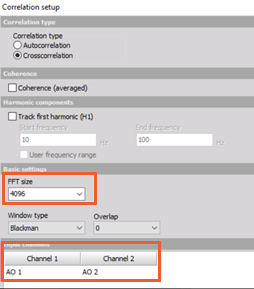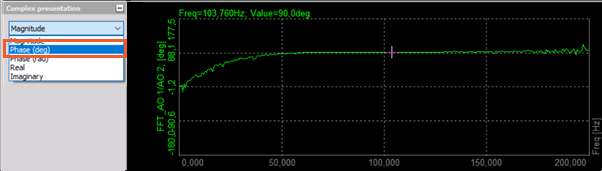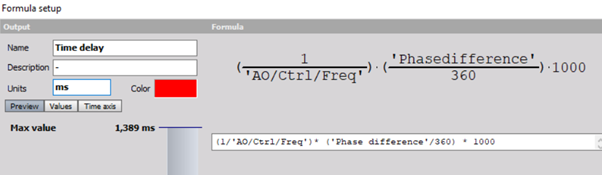For the example, we have generated two signals (sine waves) with the frequency of 100 Hz and a phase shift of 90°.

If you zoom in the signal, you can see that we expect the time delay to be 2.50 ms (calculated from 90°phase and 100Hz signal).In Correlation math set up the desired resolution and input channels:The 2D graph shows the results. The MAX crosscorrelation value is at 100 Hz:Changing the complex presentation of the crosscorrelation to PHASE(deg) will show the result:Because the max peak of crosscorrelation is at 100Hz, we are interested in the phase at 100Hz. This can be calculated with the use of the formula:This is the result:The time delay can be calculated from the phase difference and the frequency of the signal.

This calculation is shown in the formula below:Here is the result of the calculation: